# A Look Into Quantum Machine Learning

A simple overview on the current state of Quantum Machine Learning.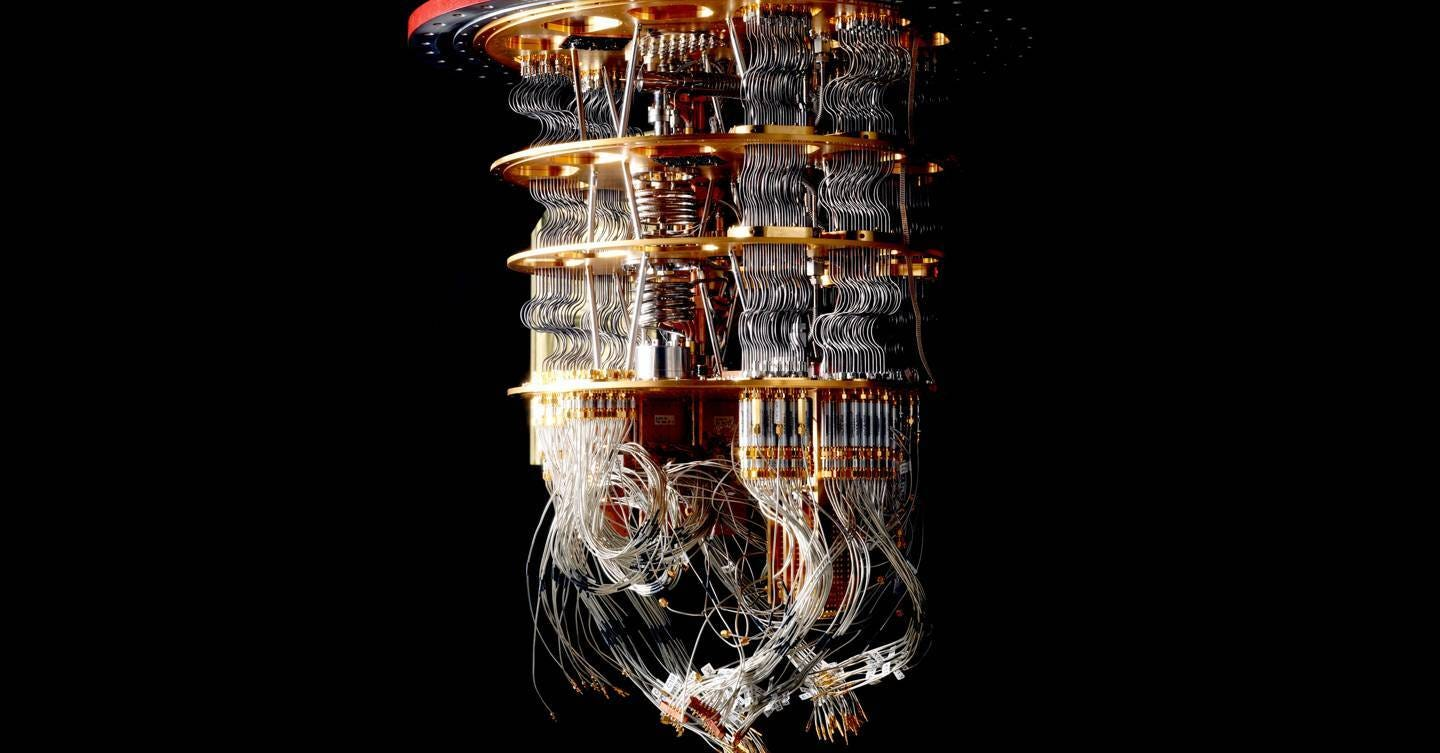Image — https://www.wired.co.uk/article/quantum-computing-explained

# State of Quantum Machine Learning

Quantum machine learning is at a rudimentary state. Research is being conducted to usefully incorporate the exponential speedup quantum computing devices promise into Machine learning. In this NISQ (Noisy Intermediate-Scale Quantum) computing era, implementing practical ML models that can give a significant edge over classical algorithms is far from being achieved! You may wonder, why is quantum computing foraying into machine learning? In a nutshell, supervised machine learning models learn probability distributions of the output. However, the intrinsic nature of Quantum Computing operations itself represents real-world probability distributions making it an ideal candidate to improve machine learning models . It can give exponential speedups using quantum properties like entanglement, superposition, interference. This makes it a lucrative option for research and finding practical quantum machine learning algorithms. In this article, we take a look into some of the progress made in QML .

## Learning in Classical Systems

Before understanding whether Quantum systems can learn we should look into how Machine Learning/Deep learning systems learn:

Most supervised Machine learning/Deep Learning (ML/DL) based systems are heuristics-based algorithms. They make use of an inner architecture (model) to represent Knowledge (Inherent features of the data) and transform them into the desired output for further processing.

E.g. — Neural networks make use of a carefully selected set of linear and non-linear transformations to map input features to labels.

## So where does the learning come in?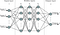Artificial Neural Network

The linear transformations are carried out using matrix multiplications and the non-linearity is created by using activation functions. Activation functions (E.g: sigmoid, tanh(x)) are used to create non-linear mappings between data points. These matrices have a set of values called weights that are randomized at the beginning of each learning cycle during training. During the initial iterations of training, these transformations on the data will most likely give a prediction with a high skew w.r.t to the expected value. The difference between these values is called the loss/error of the model and is calculated using a loss function. During each iteration of training, the weights are adjusted in such a way that the loss can be minimized by eventually reaching the global minimum. Essentially optimization (E.g: Gradient Descent) is carried out to achieve the same. This process as a whole enables learning in Classical Systems.

According to Computer Scientist Peter Wittek, who was a visionary in the field of Quantum Machine Learning :

“In any heuristics based ML model when we try to map a data point with various parameters to its corresponding label, what we are doing effectively is trying to find the conditional probability P(Label|Parameters). Hence the model is trying to find the approximate conditional probability distribution of P(L|P1, P2…PN) where P is the parameter and L is the label.”

# Can Quantum Computers Learn?

The goal of quantum computing is to harness the principles of quantum mechanics to solve problems that classical computers cannot. Hence quantum systems follow principles of quantum mechanics where ideally all closed quantum systems are reversible. Thus quantum computers transform qubits using a set of linear matrix operations called gates which are reversible in nature. These gates are unitary matrices (e.g: X, CNOT, Z, etc). Therefore a quantum circuit can be thought of as a system that takes a set of quantum states (qubits) and transforms them linearly using quantum gates . However, the aforementioned workflow of the quantum circuits poses two fundamental problems that make it hard to model a learnable system. These problems are as follows:

a) How are non-linear transformations done using Quantum gates?

b) How can these linear transformations be parameterized?

Before answering these questions let’s take a pause here and try to understand what type of Quantum Machine Learning (QML) we are looking at! The type of QML we are discussing here is using quantum processing devices for predicting/transforming/processing classical data. It is speculated that quantum machine learning using qubits (quantum data) will help us to take huge strides in this field but its applicability on near-term quantum devices is bleak, to say the least .

## Data Encoding

Before discussing how learnable quantum circuits work and are implemented, we need to encode our classical data into quantum circuits. Given the limitations of qubits available, efficient, and effective encoding is crucial. Some of the encodings used are as follows:

Amplitude Encoding: Initially a superposition of N qubits is carried out. To encode 2ᴺ data points we map these data points to the probability amplitudes of the superpositioned basis states of the qubits . E.g: to encode two sets of 4 data points a=(1.5, -2.0), b=(0.5, -2.55) we:

• Create an equal superposition of 2 qubits

1/√2(|00> + |01> + |10> + |11>)

• Map data points to the amplitude of superpositioned basis states as follows:

|00> => (1.5) * |00>

|01> => (-2.0) * |01>

|10> => (0.5) * |10>

|11> => (-2.55) * |11>

Thus we can encode exponential data using a linear amount of qubits.

Angle Encoding: This is one of the most commonly used and supposedly robust types of data encoding. Simply speaking a data point is encoded by the angle a qubit is rotated. Thus Rx/Ry/Rz gates are used to rotate a qubit by an angle θ, wherein θ is our data point being encoded. It is easy to use and intuitive .

Coming back to the question at hand,

a) How are non-linear transformations done using Quantum gates?

As non-linear Quantum gates are not reversible in nature quantum circuits don’t use them. Many problems can be mapped using linear ML models. Where a linear model is of the form:

y = a₀x₀ + a₁x₁ + a₂x₂ + … + b₀, where y is the target variable and x are the input features)

Seldom real-world problems can be mapped using linear models and most convoluted problems require a non-linear mapping (Not linearly separable/ mappable). E.g: Machine translation. Hence it seems quantum systems intrinsically cannot be used to create a learnable circuit for real-world problems. How do we tackle this problem? The non-linearity in the circuit can be imposed through the measurement gates! The measurement gates collapse the quantum states to a definite quantum state, resulting in an irreversible computation, thus giving non-linearity to the circuit .

b) How can these linear transformations be parameterized?

The aforementioned workflow does not allow us to vary the quantum operations, i.e we have a fixed set of linear operations rather than parametrized ones. However to create a learnable ML/ DL model we require a set of parameters that we can vary during the optimization stage to minimize the loss and create a universal model. How can we create such variable quantum operations? This is where Variational Quantum Circuit comes in:

# Variational Quantum Circuits to the rescue

A Variational quantum circuit is a quantum algorithm whose measurement depends on a set of free parameters θ. The quantum algorithm being modeled has a set of expectation values (a scalar) for its input qubits. The free parameters are tuned iteratively to optimize a classical cost function and get an output approximating the expected values . These are actively used in quantum chemistry, feature embeddings, HHL (model to solve a linear system of equations), etc.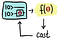Variational Quantum Circuit 

The premise of using these circuits is that they can be used like ML models wherein we can map a set of data points to definite classes. Training is done by tuning the parameters using a co-processor based classical optimization approach. These circuits can be implemented on near term quantum computers as they often learn the correct variational parameters despite the noise in the circuit. We can think of variational quantum models as a hybrid-quantum classical approach that leverages the strength of quantum computing for feature extraction/representation and classical computation for co-processor based optimization .

Simply speaking these circuits employ parameterized quantum gates to get a variational output based on the free parameters which are optimized iteratively. One example of a set of such parameterized gates is the rotation operation. These include Rx, Ry, Rz which rotate the qubit along their respective axis (x,y,z) based on the input θ . These rotation gates are used as they are highly expressive in emulating a large family of functions.

## How is the optimization carried out?

ML-based algorithms use gradient descent to minimize the cost function. Backpropagation is carried out to calculate the gradients and tune the parameters iteratively until the loss function is minimized. As variational circuits also make use of classical optimization techniques the only problem that remains is how to calculate gradients of a Quantum Circuit. These quantum gradients are calculated using the parameter shift rule . To calculate the gradient:

• Shift the parameter of the circuit θ in both the positive and negative direction

Up: U(θ+x)

Down: U(θ-x)

• The difference between these values is the effective gradient

∇ = 1/2( U(θ+x) - U(θ-x) )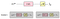Parameter Shift Rule 

We can calculate this gradient without knowing the inner workings of the circuit. Once this gradient is calculated the optimization is carried out as usual. Variational models also suffer from the issue of barren plateaus. This area is currently under research and some of the solutions suggested are using a predefined parameter initialization or using a local cost function. Now that we have got an idea of the working and importance of variational circuits in QML, let’s look at some Variational architectures used in QML.

# Quantum Feature Maps

Various machine learning models transform input data to different feature spaces for feature extraction/classification or further processing. The function Φ used to do this transformation is called a feature map. The crux behind using such a mapping is to make it easier for the segregation of data points in a proper higher-dimensional space. Essentially hidden layers in a neural network up to the last layers extract features/information which can easily be used by the final layer for classification/ regression etc .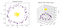Feature Map 

Support vector machine (SVM) is defined by Analytics Vidhya as follows :

“In the SVM algorithm, we plot each data item as a point in n-dimensional space (where n is the number of features you have) with the value of each feature being the value of a particular coordinate. Then, we perform classification by finding the hyper-plane that differentiates the two classes very well”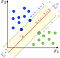SVM 

The maximal margin hyperplane (boundary to separate data points according to classes) in a support vector machine implicitly depends on the inner product of the data points being classified. To segregate non-linear data SVM implicitly transforms the data points to a higher dimension where the data will be linearly separable. Explicitly transforming data to a higher dimension is computationally expensive/impossible. Rather than transforming the data points directly to a higher dimension, the kernel function uses the kernel trick to calculate the inner product of the data points in the higher dimension easily. This sum of inner products is used to find the non-linear boundary/hyperplane to separate the non-linear data.

To understand how a support vector machine works and how the maximal classification boundary is calculated, refer to this link:

During the data encoding/state preparation phase classical data is encoded into the quantum circuit using various algorithms. In angle encoding data is encoded as the angle of the rotation gates. This encoding essentially maps the classical data into a higher dimension of an exponentially large Hilbert space. Thus a linear gate can transform data in a non-linear fashion to a higher data plane. Thus encoding classical data into quantum states is considered a quantum feature map .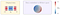Data Embedding in Hilbert Space 

## Quantum Kernel Function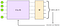Quantum Kernel 

In a Support Vector Machine, the maximal margin boundary is calculated implicitly using the kernel trick as mentioned above. Thus the most important step in SVM is calculating the sum of the inner products of the data points in a higher dimension by using the kernel trick, as once that is found we can calculate the hyperplane separating the non-linear data easily. We know that angle encoding creates a non-linear transformation to Hilbert space. So we can use a variational circuit to map this data in Hilbert space to the inner product of the data points, i.e we can train the variational circuit to map input data points to their inner product. Thus we are parameterizing the embedding function itself to create a variational circuit that outputs the inner-product of data points. Then classical optimization is carried out to minimize the loss in this mapping. SVM that uses this kernel is called a Quantum Enhanced SVM .

# Quantum Neural Networks (QNN)

Neural networks form the basis of many deep learning models. They enjoy widespread use in the industry and research. Creating quantum circuits analogous to Neural networks is a key step for progressing and finding the limits of Quantum Machine Learning. Below is the circuit diagram of a general design of a QNN circuit.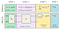QNN General Architecture 

As we can see from the circuit, we have the starting states initialized to zero. Now we do state preparation where Quantum embedding is applied to encode classical data into a quantum circuit using angle encoding. In the next step, we perform a linear transformation which is equivalent to a weighted sum of the inputs in a feed-forward neural network (i.e it acts as a linear layer). Then for the last layer, after a series of linear operations, we perform a measurement gate to apply non-linearity to the circuit and measure the probabilistic scalar output. This operation is applied last as it is unnatural and not possible to apply a series of activation functions in between the linear transformations for a quantum circuit. Finally, the output is feed to a classical optimization circuit. It will iteratively calculate the loss, use gradient descent, update the parameters till a global minimum is reached . We have just gone through the basic design of a QNN Circuit.

## Variational Quantum Classifier (VC)

A Variational Quantum classifier can be thought of as a type of QNN although both terms are used interchangeably. The inherent architecture is same as the QNN. The goal of this QML model is to classify data points. Classical data is encoded using angle encoding. As discussed above this encoding maps the data to a higher-dimensional Hilbert space. Here it will be easier to separate the data. Then the variational circuit which is a set of linear layers is applied. Its goal is to create a hidden layer-based architecture that can map the data points in the Hilbert space to their respective classes. Initially, this mapping will be randomized but iteratively learned by the variational circuit.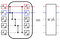Variational Model Architecture 

Various types of variational circuits can be used depending upon the problem/circuit to maximize the expressibility (Range of data that can be mapped) of the QNN. All of these have the same inherent structure wherein a set of rotation gates are applied to the qubits followed by an entanglement of all the qubits, and then by some more rotation gates. This circuit can be repeated to increase the depth of the neural network and increase the complexity of the transformations. The entanglements are acted upon to increase expressibility, complexity, and depth of the model using quantum properties. Finally, measurement is conducted to map the output probability distribution to a binary output class. This output is supplied to the classical optimization function to minimize the mapping loss and create a classification model .

# QML in Q#

The QML library is a recent addition to QDK (Quantum Development Kit). It provides the means to implement the Variational Quantum Classifier using Q#. The documentation provides a set of tutorials that cover all the concepts from basic to advance for designing our own classifier using a custom dataset. This blog gives a simple introduction to QML, thus for a more hands-on approach complete the tutorials and read the documentation.

The link to the documentation is as follows:

# Future prospects for QML

Quantum machine learning is still in its dormant stages. It is a subject heavily under research, and many of the concepts are still being formulated in an ever-changing landscape. Research is being carried out to utilize the interesting properties of Quantum Computing in Machine learning and create an altogether different architecture or have exponential speedups in current algorithms. Some propositions are looking to embed quantum neural layers in some deep learning-based models for rich feature extraction and increasing expressibility of the circuit. The possibility of creating quantum GAN’s for label prediction of a vast amount of unlabelled data is being investigated . The direction QML is heading seems fascinating to me. Pragmatically speaking there is currently no useful QML model that can replace current ML/DL ones. However, in the future once utilized to its full potential, it can shake the landscape of traditional computing. What do you think? Write your thoughts in the comments below!

This post is part of the Q# Advent Calendar 2020. Follow the calendar for other great posts!

# Picture Credits:

Hey I am Kunal from India. I work in Wipro for the Blockchain Research team. My main interests are in Blockchain, ML, Competitive programming and Cybersecurity.

## More from Kunal Kasodekar

Hey I am Kunal from India. I work in Wipro for the Blockchain Research team. My main interests are in Blockchain, ML, Competitive programming and Cybersecurity.